# Maths

Mathematics concepts: Maths is not only a subject taught in school but also has several applications in real life. Some kids find maths easy while some find it difficult. Parents find it a challenge to make kids love Maths. It takes lots of practice to master Maths. Some students may find Maths hard to understand and implement. Thus, GeeksforGeeks presents here a simple way for kids and students to learn Maths in a  hassle-free manner.

Online Maths learning for students for free has been brought at GeeksforGeeks for students so that they can easily learn and understand each fundamental basic Maths concepts. We have provided Mathematics learning materials for all standards in this article.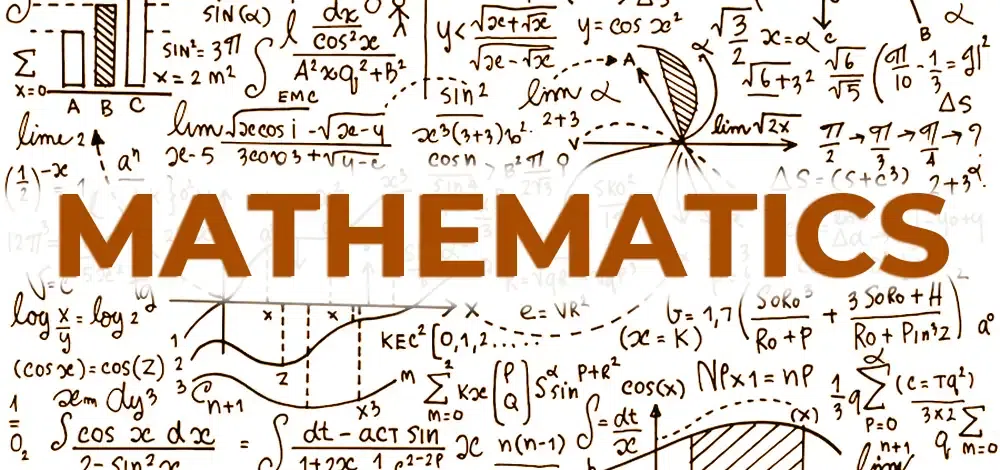## Maths Mapping

In mapping Maths, we acknowledge several concepts of Mathematics. The origin or base of Maths is Counting, where we count the objects we see. Mathematics can be divided into two parts:

• Pure Mathematics (number system,geometry, matrix,algebra,combinatorics,topology,calculus)
• Applied Mathematics (Engineering, Chemistry, Physics, Numerical Analysis, etc).

Maths has several concepts; to solve problems on those concepts, we need to understand those concepts and learn the formulas related to different concepts. You can find the Maths formulas related to all concepts.

## Maths Online

There are various simple and advanced concepts in Mathematics. These concepts are taught to students according to their difficulty and complexity level.  Basic concepts like numbers, addition, subtraction, multiplication, division, place value, etc are taught to kids first, and later enhanced concepts such as ratios, percentages, algebra, trigonometry, mensuration, etc are taught. Advanced Concepts like Integration and differentiation, Permutation and combination, and Probability, are included in secondary school.

You can find the class-wise Maths syllabus prepared by our GeeksforGeeks expert in the given links.

## Branches of Mathematics

### Pure Mathematics

This part of mathematics is divided into 6 major sections:

### Number Theory

Number theory is an area of mathematics that studies the set of positive whole numbers, such as 1, 2, 3, 4, 5, 6, etc., known as natural numbers.
Number theory makes the connections between various types of numbers easier. In number theory, numbers have been divided into different categories such as:

• Odd Numbers – 1, 3, 5, 7, 9, 11, 13, 15, 17, 19…..
• Even Numbers – 2, 4, 6, 8, 10, 12, 14, 16, 18, 20, 22 . . .
• Square Numbers – 4, 9, 16, 25, 36, 49, 64, 81,100 . . .
• Cube Numbers – 8, 27, 64, 125, 216, 343, 512 . . .
• Prime Numbers – 2, 3, 5, 7, 11, 13, 17, 19, 23, 29, 31, 37, 41, 43, 47,53, 59, 61 . . .
• Composite Numbers – 4, 6, 8, 9, 10, 12, 14, 15, 16,18, 20, 21, 22, 24 . . .
• 1 (modulo 4) Numbers – 1, 5, 9, 13, 17, 21, 25, . . .
• 3 (modulo 4) Numbers – 3, 7, 11, 15, 19, 23, 27, . . .
• Triangular Numbers – 3, 6, 10, 15, 21, 28, 36, 45,. . .
• Perfect Numbers – 6, 28, 496, 8128, . . .
• Fibonacci Numbers -1, 1, 2, 3, 5, 8, 13, 21, 34, 55, 89. . .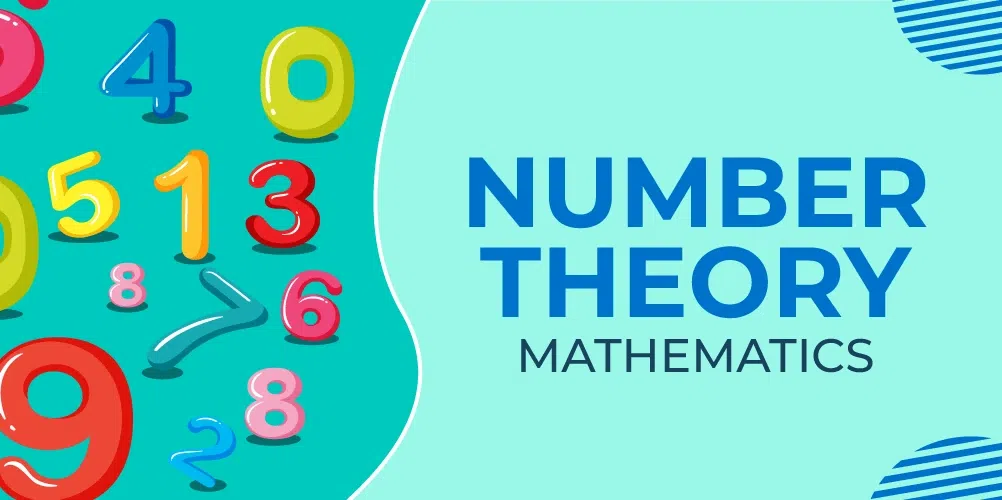Key Concepts

• Number System: Gain a comprehensive understanding of the Number System in Maths, covering its fundamental concepts and types.
• Numbers: Explore the world of Numbers, their classifications, and properties.
• Prime Numbers: Delve into the intriguing world of Prime Numbers and their significance in mathematics.
• Real Numbers: Understand the concept of Real Numbers, their characteristics, and applications.
• Rational Numbers: Learn about Rational Numbers and their place in the number system.

### Algebra

Algebra is the area of mathematics that helps represent conditions or problems into mathematical expressions. These expressions include variables like x,y, and z and operations like addition, subtraction, multiplication, and division implemented on them.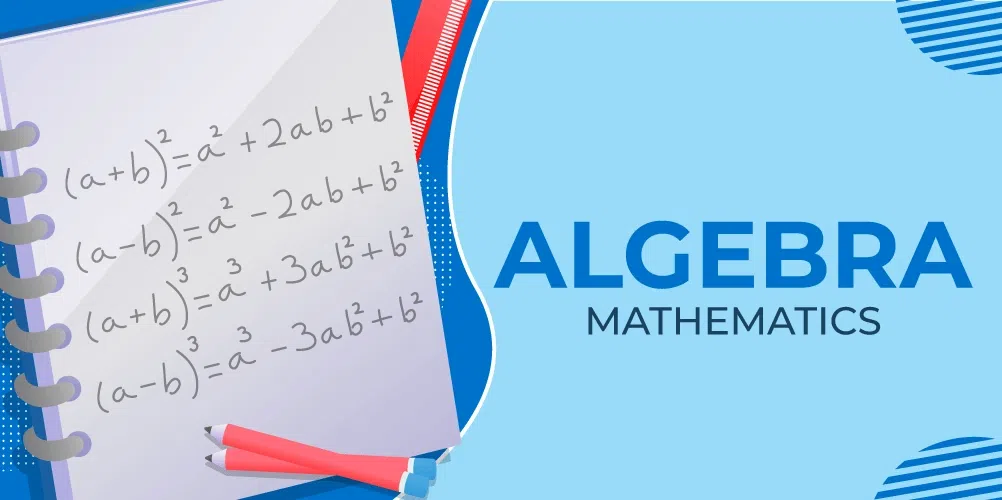Key Concepts

### Geometry

Geometry is the Mathematics section concerned with the dimensions, sizes, angles, and forms of a wide range of items we see every day. There are various types of geometry such as plane geometry, three-dimensional geometry, solid geometry, etc.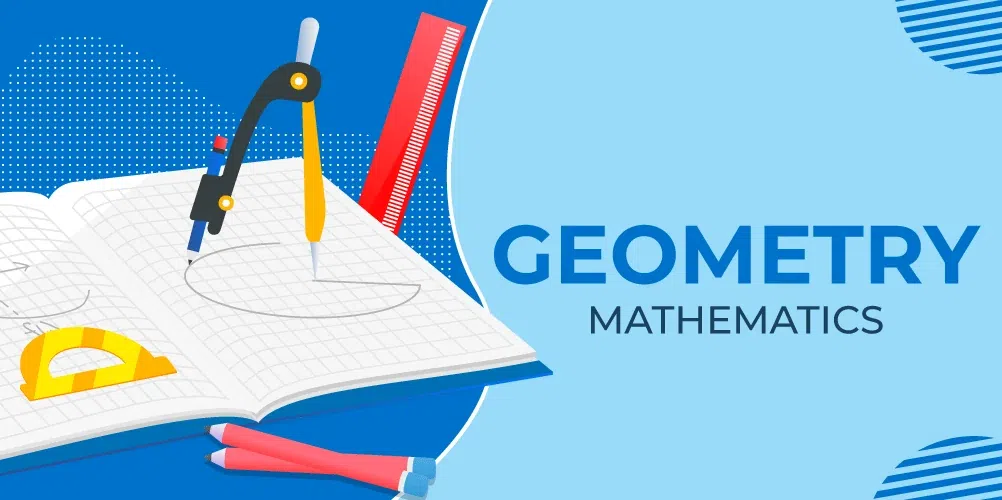Key Concepts

• Geometric Shapes: Get acquainted with various Geometric Shapes.
• Types of Polygons: Explore the Types of Polygons and their properties.
• Triangle: Learn about the properties and types of Triangles.
• Circle: Delve into the properties and equations of Circles.
• Types of Angles: Familiarize yourself with the Types of Angles in geometry.

### Arithmetic

Arithmetic is a branch of mathematics that includes the operations of numbers. This field of mathematics serves as the foundation for students to understand mathematics and implement it in real life.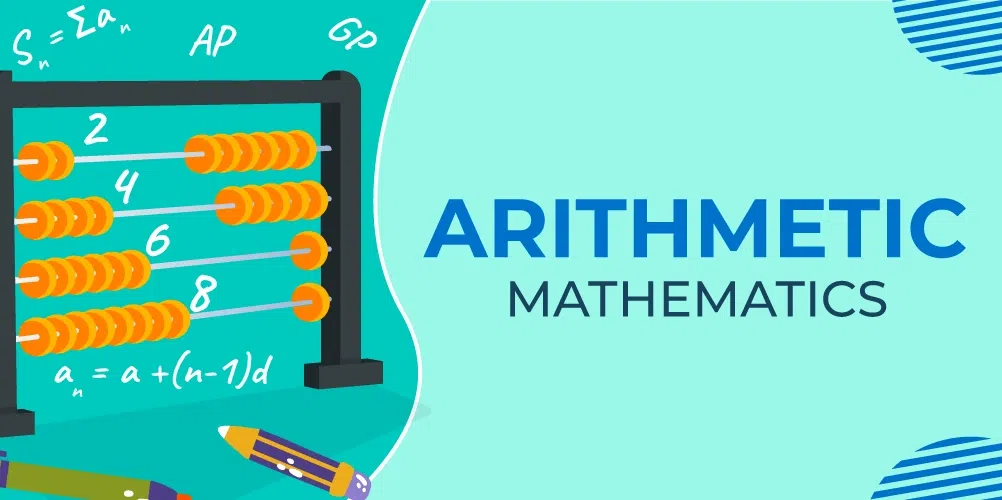Key Concepts

• What is Arithmetic: Learn about the basics of Arithmetic and its importance.
• Arithmetic Operations: Familiarize yourself with various Arithmetic Operations.
• Division: Understand the process and rules of Division.
• Arithmetic Progression: Explore the concept of Arithmetic Progression and its applications.

### Combinatorics

A branch of mathematics called combinatorics is concerned with the investigation of finite discrete structures. Enumerations of the sets of elements and the study of permutations and combinations are covered. It describes the characteristics of mathematical relations.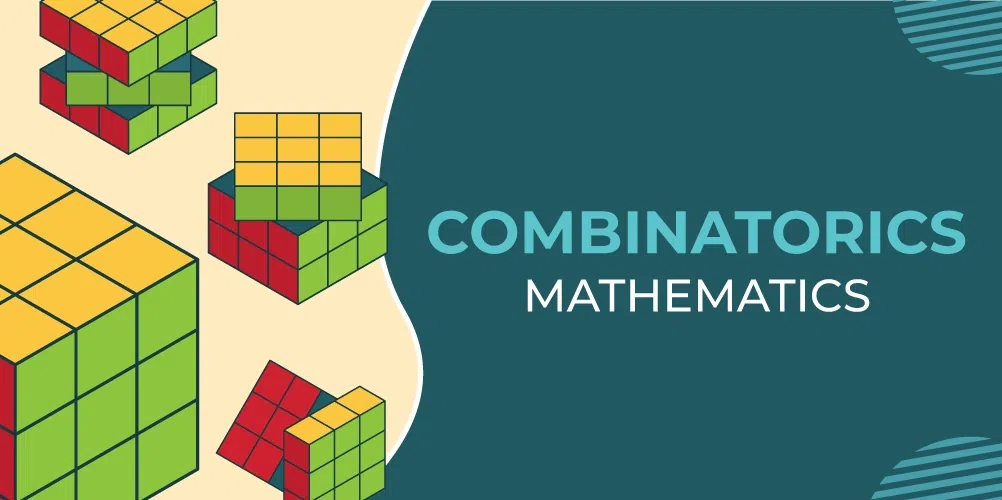Key Concepts

### Topology

Topology studies properties of spaces that are invariant under any continuous deformation. Because the objects may be stretched and contracted like rubber sheets yet cannot be broken, it is frequently referred to as “rubber-sheet geometry”.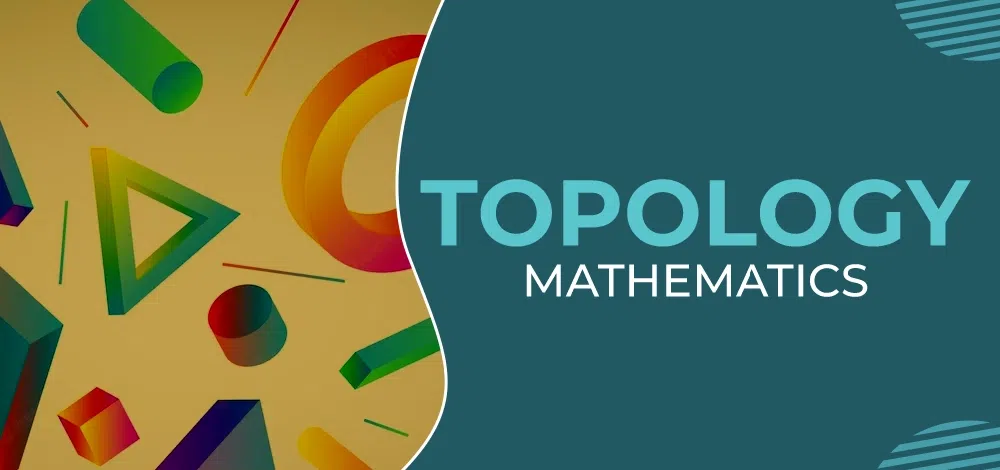### Mathematical Analysis

The area of mathematics known as analysis is concerned with continuous functions, limits, and associated theories, including measure, differentiation, integration, infinite sequences, and series, as well as analytical functions.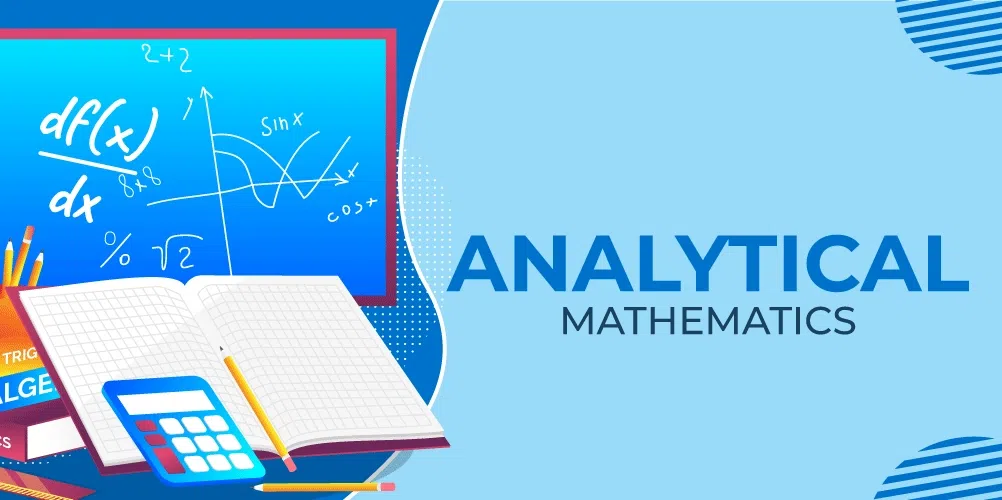### Applied Mathematics

This part of mathematics is divided into 4 major sections:

### Calculus

Calculus is a field of mathematics that is concerned with the computation of instantaneous rates of change (differential calculus) and the summing of an infinite number of minor elements to find a single whole.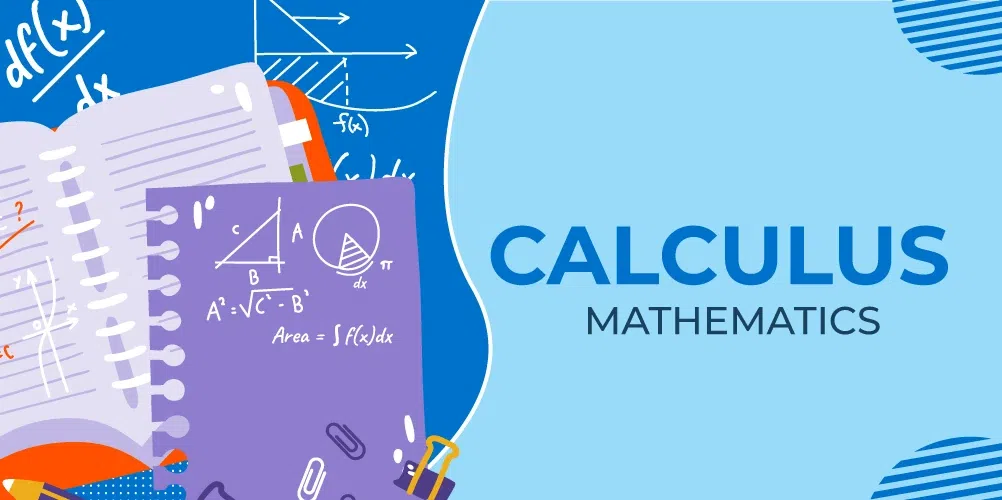Key Concepts

### Statistics and Probability

The two most crucial ideas in mathematics are probability and statistics. Probability is mainly about chance, and statistics, on the other hand, is about how we manage varied data using various methods. It enables in the easy and clear simplification of very complex data. We discuss the probability of various outcomes if we are unsure about how an event will turn out. Statistics is the study of these probabilistically determined occurrences.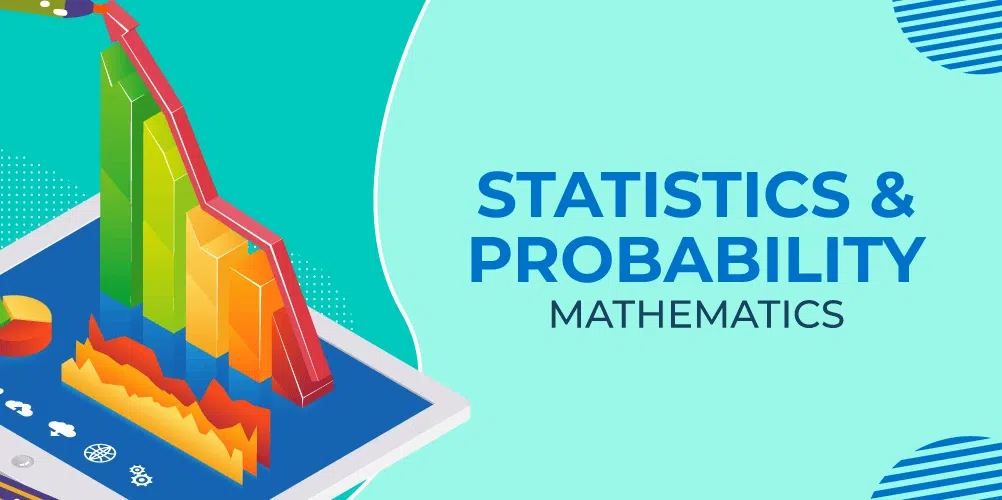Key Concepts For Statistics,

• Central Tendency: Learn about measures of Central Tendency.
• Frequency Distribution: Explore the concept of Frequency Distribution.
• Standard Deviation: Understand the calculation and significance of Standard Deviation.
• Mean: Discover how to calculate the Mean of a dataset.
• Median: Learn about finding the Median in statistics.
• Mode: Understand what the Mode is and how to find it.

Key Concepts For Probability,

### Set Theory

This branch of mathematics studies sets, which are basically the collection of things or objects or data.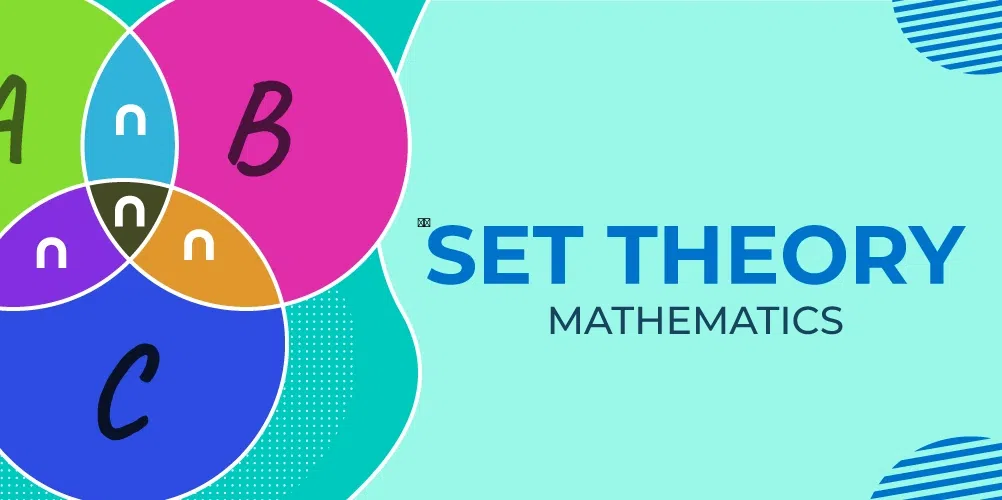Key Concepts

### Trigonometry

Trigonometry is the field of mathematics that deals with certain angles’ functions and how to use such functions in computations. There are six popular trigonometric functions for an angle.

• Sine (sin)
• Cosine (cos)
• Tangent (tan)
• Cotangent (cot)
• Secant (sec)
• Cosecant (csc)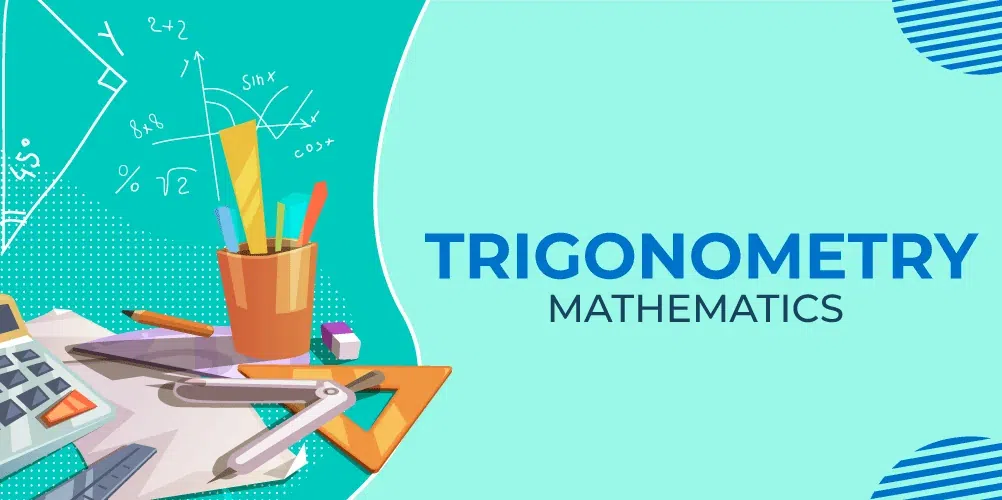Key Concepts

## Maths Topics

Here is a list of important topics in Maths, you can click on the topic to learn the topic directly:

 Area of Circle Area of Rectangle Area of Triangle Arithmetic Progressions Ascending Order Bayes Theorem Binomial Calculus Cartesian Coordinate System Cartesian Product of Sets Circles Conic Section Construction Continuity and Differentiability Cube and Cube Root Data Organization Determinants and Matrices Even Numbers Factorisation Of Polynomial Greatest Common Factor HCF and LCM Heron’s Formula Integration Laplace transform Linear Programming Mathematical Induction Natural Numbers Negative Exponents Number System Odd Numbers Permutation and Combination Playing with Numbers Polynomials Prime Number Quadratics Quadrilateral Rational Numbers Ratio and Percentages Real Numbers Sequence and series Sets Slope of Straight Line Square and Square Roots Surface Area and Volume The perimeter of a triangle Triangles Trigonometry Table Value of Pi Vector Algebra Whole Numbers

## How to learn Maths?

Maths is a simple as well as a tricky subject. Maths is fun to explore but tough to excel. At GeeksforGeeks, you can clear all fundamental concepts and build a strong foundation in this subject. When you get a good grip and clear understanding of this subject, your brain will think logically and help you grasp the concepts in all other subjects with excellent efficiency.

We are providing learning materials for students of Class 8 to 12 based on the latest syllabus approved by CBSE. In these classes, Maths for students understand the important concepts of Maths, like basic geometry, decimals, algebra, ratio, and proportions, etc. Our experts at GeeksforGeeks have curated the concepts in such an interactive manner for the students which helps students to grasp the basics of any topic in the most efficient way possible.

With the help of animations and creative ways, our experts have tried their level best to ensure that the students in the best way possible understand concepts. The sole purpose is to make maths easy to learn and implement the concepts with ease when solving problems.

## Basic Maths

The basics of Maths involve arithmetic operations such as :

• Subtraction(-)
• Multiplication(x)
• Division(/)

To grasp these basic concepts most efficiently, Maths for students need to practice questions and solve worksheets based on them. Concepts like HCF and LCM, multiples, and factors, will be introduced to students in their secondary classes.

## Conclusion: The Importance of Learning Mathematics

In conclusion, the study of mathematics is not just a pursuit for academic excellence, but a fundamental tool that equips us to navigate the complexities of the world. Mathematics is the language of logic and precision, and its mastery is essential for a variety of fields, from engineering and technology to finance and the natural sciences.

Understanding mathematics enhances our problem-solving skills, enabling us to analyze and interpret data, and make informed decisions. It fosters critical thinking and analytical skills, which are invaluable in both professional and personal contexts. The principles of mathematics underpin the advancements in technology and science, driving innovation and progress.

Furthermore, mathematics plays a crucial role in our daily lives. From managing finances and understanding statistical information presented in the media, to appreciating the beauty of patterns and structures in nature, mathematics is omnipresent. It empowers us to understand and appreciate the world in a more structured and logical manner.

## FAQs on Mathematics Learning

### Q1: Why is it important to learn mathematics?

Mathematics is essential for developing problem-solving and analytical skills. It’s fundamental in various fields like science, engineering, and technology, and helps in making informed decisions in everyday life..

### Q2: Can mathematics help in other subjects?

Absolutely. Mathematics enhances logical reasoning and analytical thinking, skills that are beneficial in subjects like physics, chemistry, economics, and even in understanding complex patterns in languages.

### Q3: How can I improve my mathematics skills?

Regular practice is key. Focus on understanding concepts rather than just memorizing formulas. Utilize resources like GeeksforGeeks for guided learning, and don’t hesitate to seek help when needed.

### Q4: Is mathematics only about numbers and calculations?

No, mathematics is much more. It includes understanding patterns, logical reasoning, abstract thinking, and problem-solving, which go beyond mere numbers and calculations.

### Q5: How can parents help their children in learning mathematics?

Parents can encourage a positive attitude towards math, help with regular practice, and use real-life examples to make math more relatable and interesting for their children.

### Q6: Are online resources effective for learning mathematics?

Yes, online resources like interactive tutorials, videos, and problem-solving exercises can be very effective, especially when they complement traditional learning methods.

### Q7: What are the major branches of mathematics covered in this guide?

This guide covers various branches including Algebra, Geometry, Calculus, Trigonometry, Probability, and more, catering to a wide range of learners.

### Q8: Is mathematics essential for higher education?

Yes, a strong foundation in mathematics is crucial for higher education, especially for courses in science, technology, engineering, and mathematics (STEM) fields.

### Q9: Can learning mathematics help in career development?

Definitely. Many careers require strong mathematical skills, including engineering, data science, finance, and research. Proficiency in math can open up numerous career opportunities.

### Q10: How can I make mathematics learning more engaging?

Try to apply mathematical concepts to real-life problems, engage in mathematical games and puzzles, and use interactive learning tools available on platforms like GeeksforGeeks.

Whether you're preparing for your first job interview or aiming to upskill in this ever-evolving tech landscape, GeeksforGeeks Courses are your key to success. We provide top-quality content at affordable prices, all geared towards accelerating your growth in a time-bound manner. Join the millions we've already empowered, and we're here to do the same for you. Don't miss out - check it out now!